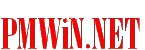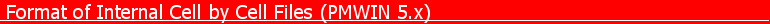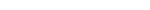Welcome! [Home] [PMWIN] [3D Visualization] [ASMWIN] [Downloads] [Links]by Wen-Hsing Chiang Last Update: December 05, 2003This file describes the internal cell-by-cell binary files of the version 5.0 of PMWIN. Here is the source code (in Visual Basic 5) for reading and saving the cell-by-cell files 1. GRID GEOMETRY: The grid geometry is saved in the fn.XY file, where fn is the file base of a PM5-MODEL file. For example, if a PM5-MODEL is C:\PM5\DATA\EXAMPLE.PM5, the grid geometry is saved in the C:\PM5\DATA\EXAMPLE.XY file. File Format: NX, NY, NZ DX(1) DX(2) . . DX(nx) DX(1) DX(2) . . DX(ny) Explanation of Fields Used in Input Instructions NX is the number of model columns. NY is the number of model rows. NZ is the number of model layers. DX is the cell width along rows. This is a single array with one value for each column. DY is the cell width along columns. This is a single array with one value for each row. 2. TIME-INDEPENDENT MODEL DATA FILES Time-indenpendent model data (such as hydraulich conductivity or porosity) are saved in time-independent model data file, which has a (dummy) stress period header of 1000*2 bytes and a (dummy) position header of 1000*2 bytes. The header are not used here. Following the headers, PMWIN uses REAL (4 bytes) to save cell-by-cell model data seguentially. The following example illustrates a model data file with NJ columns, NI rows and 2 layers. ``` STRESS PERIOD HEADER (1000*2 bytes) POSITION HEADER (1000*2 bytes) f(1,1) f(1,2) ... f(1,J) .... f(1,NJ) --- f(2,1) f(2,2) ... f(2,J) .... f(2,NJ) | . . . . | 2D matrix for layer 1 . . . . | NI*NJ*4 bytes f(I,1) f(I,2) ... f(I,J) .... f(I,NJ) | . . . . | . . . . | f(NI,1) f(NI,2)... f(NI,J) ....f(NI,NJ) --- f(1,1) f(1,2) ... f(1,J) .... f(1,NJ) --- f(2,1) f(2,2) ... f(2,J) .... f(2,NJ) | . . . . | 2D matrix for layer 2 . . . . | NI*NJ*4 bytes f(I,1) f(I,2) ... f(I,J) .... f(I,NJ) | . . . . | . . . . | f(NI,1) f(NI,2)... f(NI,J) ....f(NI,NJ) ---``` 3. TIME-DEPENDENT MODEL DATA FILES A time-dependent model data file has two headers STRESS PERIOD HEADER and POSITION HEADER. Each header has 1000 * 2 bytes (Integers). Each integer of the STRESS PERIOD HEADER corresponds to a stress period (max. 1000 stress periods are allowed). If the i-th integer has the value j, the data of the j-th period will be used for the i-th stress period. The value i of the j-th integer of the POSITION HEADER indicates that the data of the j-th stress period is saved in the i-th position of the file. This data structure allows a user to jump between stress periods in the Data Editor without having to save data of all stress periods. In the following example, a model has 2 layers and 8 stress periods. According to the STRESS PERIOD HEADER, the data specified for the first stress period will be used for the 1st and 2nd periods. The data specified for the 3rd stress period will be used for the periods 3 and 4. The data specified for the 5-th stress period will be used for the periods 5, 6, 7 and 8... According to the POSITION HEADER, the data of the 1st, 3rd and 5th stress periods are saved in the position 1, 3 and 2, respectively. ```STRESS PERIOD HEADER ------> 1 2 3 4 5 6 7 8 .... (1000 * 2 bytes) Integer values: 1 1 3 3 5 5 5 5 .... |__| |________| | | | | POSITION HEADER -----------> 1 0 3 0 2 0 0 0 0 .... (1000 * 2 bytes) | | | |________________________ |_________________________________ | | | position 1: 2D-matrix for layer 1, period 1 <-| | (begin: 4001 bytes) 2D-matrix for layer 2, period 1 <-| | | position 2: 2D-matrix for layer 1, period 5 <----| (4001+(2-1)*NI*NJ*4) 2D-matrix for layer 2, period 5 <----| position 3: 2D-matrix for layer 1, period 3 (4001+(3-1)*NI*NJ*4) 2D-matrix for layer 2, period 3 ```